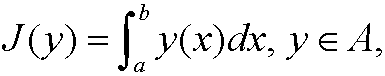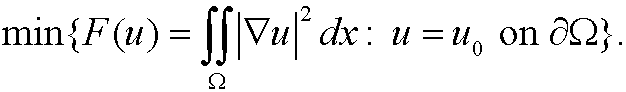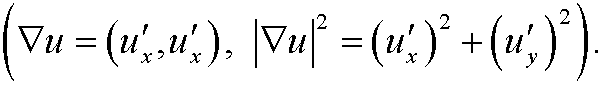4. Some examples of variational problems.

Example 4: Let A be the setFind minimum to the functional J(y) whenRemark: This functional describes the curve length between the points (a,y0) and (b,y1) (see the figure below).Example 5 (The Brachistochrone problem), from Greek brachistos(shortest) and chronos (time)): A particle with mass m starts from rest and glides without friction under the influence of gravity from the point (x1,y1)to the point (x2,y2) along the curve y=y(x) in the xy-plane. What curve gives the shortest time of descent? (Bernoulli 1696).Since the speed along the curve is v=ds/dt, we have for the total time of descent thatTo compute v as function of the coordinates we use that a decrease of potential energy is followed by an equally large increase of kinetic energy assuming that we do not have any friction. If the acceleration of gravity is g, this implies thatthat is, the speedv(x) is given byThis leads to the variational problem of minimizingwhenExample 6: Minimize the functionalwhenWhat is the interpretation of this problem and what is the solution?
Example 7: Let A be an arbitrary function space on [a,b]. Thenis also a functional.
Example 8 (the soap film problem, Plateau’s problem): FindRemark: This functional describes the area of a surface that is spanned by the curve u0 on the boundary to. The soap film we get by dipping a shaped wire in soap solution will fulfill the above variational problem. The figure below shows how a soap film on an elastic ring will change as the ring is stretched to a parabolic shape (in the x-direction).Example 9 (Dirichlet’s integral, Principle of least work): FindRemark: